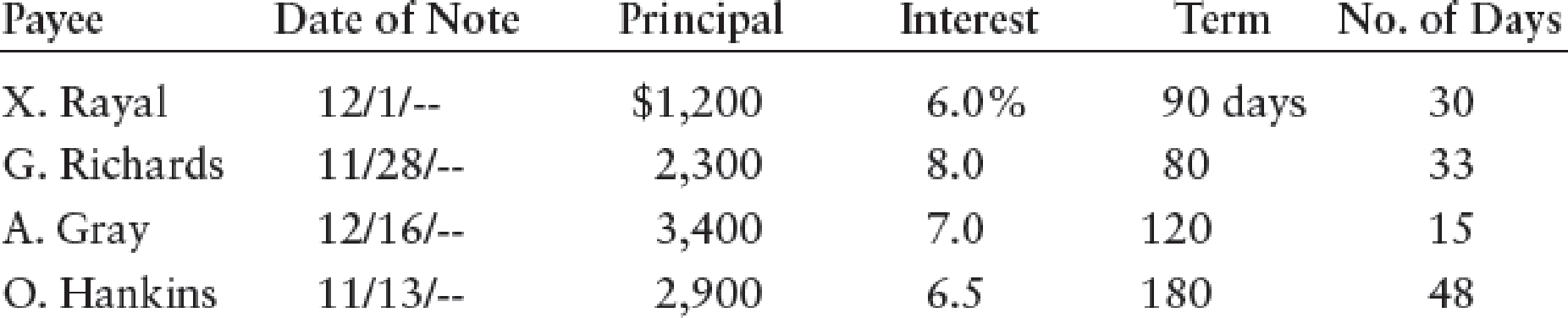Chapter 17, Problem 14SPB

Chapter
Section
Textbook Problem

ACCRUED INTEREST PAYABLE The following is a list of outstanding notes payable as of December 31, 20--:REQUIRED 1. Compute the accrued interest at the end of the year. 2. Prepare the adjusting entry in the general journal.

1.

To determine

Compute the accrued interest at the end of the year.

Explanation

Accrued interest:

Accrued interest is the outstanding interest expense in the accounting period, as on the reporting date of financial statements.

 Maker Principal (A) Interest (B) Time (C ) Interest ((A × B × C) ÷ 360 Person X.R \$1,200 6% 30 \$6...

2.

To determine

Prepare the adjusting entry of accrued interest payable.

Still sussing out bartleby?

Check out a sample textbook solution.

See a sample solution

The Solution to Your Study Problems

Bartleby provides explanations to thousands of textbook problems written by our experts, many with advanced degrees!

Get Started

Find more solutions based on key concepts